# Maharashtra Board Class 6 Maths Solutions Chapter 17 Geometrical Constructions Practice Set 40

## Maharashtra State Board Class 6 Maths Solutions Chapter 17 Geometrical Constructions Practice Set 40

Question 1.
Draw line l. Take point P anywhere outside the line. Using a set square draw a line PQ perpendicular to line l.
Solution:
Step 1:Step 2: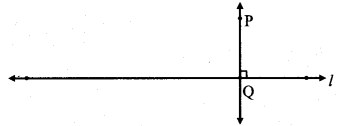line PQ ⊥ line l.

Question 2.
Draw line AB. Take point M anywhere outside the line. Using a compass and ruler, draw a line MN perpendicular to line AB.
Solution:
Step 1: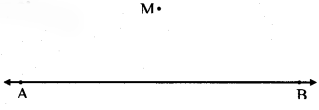Step 2:Step 3: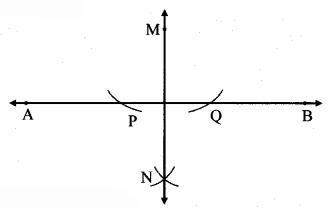line MN ⊥ line AB.

Question 3.
Draw a line segment AB of length 5.5 cm. Bisect it using a compass and ruler.
Solution:
Step 1: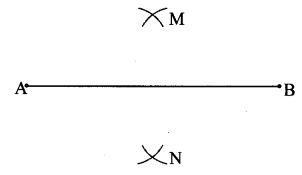Step 2:line MN is the perpendicular bisector of seg AB.

Question 4.
Take point R on line XY. Draw a perpendicular to XY at R, using a set square.
Solution:
Step 1: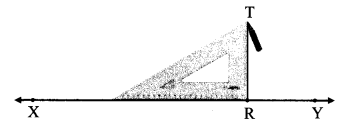Step 2:line TR ⊥ line XY.

#### Maharashtra Board Class 6 Maths Chapter 17 Geometrical Constructions Practice Set 40 Questions and Activities

Question 1.
In the above construction, why must the distance in the compass be kept constant? (Textbook pg. no. 90)
Solution:
The point N is at equal distance from points P and Q.
If we change the distance of the compass while drawing arcs from points P and Q, we will not get a point which is at equal distance from P and Q. Hence, the distance in the compass must be kept constant.

Question 2.
The Perpendicular Bisector. (Textbook pg. no. 90)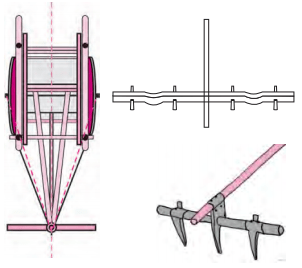1. A wooden ‘yoke’ is used for pulling a bullock cart. How is the position of the yoke determined?
2. To do that, a rope is used to measure equal distances from the spine/midline of the bullock cart. Which geometrical property is used here?
3. Find out from the craftsmen or from other experienced persons, why this is done.

Solution:

1. For the bullock cart to be pulled in the correct direction by the yoke, its Centre O should be equidistant from the either sides of the cart.
2. The property of perpendicular bisector is used to make the point equidistant from both the ends
3. A rope is used just like a compass to get equal distances from the spine/midline of bullock cart.

Question 3.
Take a rectangular sheet of paper. Fold the paper so that the lower edge of the paper falls on its top edge, and fold it over again from right to left. Observe the two folds that have formed on the . paper. Verify that each fold is a perpendicular bisector of the other. Then measure the following distances. (Textbook pg. no. 91)
i. l(XP)
ii. l(XA)
iii. l(XB)
iv. l(YP)
v. l(YA)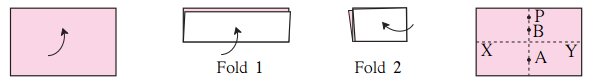You will observe that l(XP) = l(YP), l(XA) = l(YA) and l(XB) = l(YB)
Therefore we can conclude that all points on the vertical fold (perpendicular bisector) are equidistant from the endpoints of the horizontal fold.
Solution:
[Note: Students should attempt this activity on their own.]